## BUFFER AND COMPLIANT DYNAMIC SURFACE CONTROL OF SPACE ROBOT CAPTURING SATELLITE BASED ON COMPLIANT MECHANISM$^{\bf 1)}$

School of Mechanical Engineering and Automation, Fuzhou University, Fuzhou 350116, China

 基金资助: 1)国家自然科学基金.  11372073国家自然科学基金.  51741502福建省工业机器人基础部件技术重大研发平台.  2014H21010011

Received: 2019-03-5   Accepted: 2020-04-1   Online: 2020-07-18Abstract

The buffer and compliant control for space robot to avoid joint damage during on-orbit capture non-cooperative satellite are studied. For the reason, a compliant mechanism is mounted between the joint motor and space manipulator, its functions are: first, the deformation of internal spring in compliant mechanism can absorb the impact torque of the captured satellite acting on the joint of the space robot; second, the joint impact torque can be limited to a safe range by reasonably designing the buffer and compliant control scheme. First of all, the dynamic models of the space robot system and the target satellite system before capture are derived by multi-body theory. After that, based on the law of conservation of momentum, the constraints of kinematics and the law of force transfer, the integrated dynamic model of the combined system is derived. At the same time, the impact effect and impact force are calculated. For the stabilization control of post-capture unstable combined system, a buffer and compliant control scheme based on dynamic surface is proposed. The proposed control scheme can not only effectively absorb the impact torque generated by the on-orbit capture process, but also timely open or close the joint motor when the impact torque is too large, which can avoid overload and damage of the joint motor. In addition, the dynamic surface control scheme is utilized to avoid calculation expansion caused by backstepping method and to reduce the calculation effectively. The stability of the system is proved by Lyapunov theorem, and numerical simulation verifies the effectiveness of the proposed buffer and compliant control method.

Keywords： compliant mechanism ; space robot ; capture satellite operation ; buffer and compliant control ; dynamic surface control

Ai Haiping, Chen Li. BUFFER AND COMPLIANT DYNAMIC SURFACE CONTROL OF SPACE ROBOT CAPTURING SATELLITE BASED ON COMPLIANT MECHANISM$^{\bf 1)}$. Chinese Journal of Theoretical and Applied Mechanics[J], 2020, 52(4): 975-984 DOI:10.6052/0459-1879-20-068

## 1 柔性机构结构及缓冲从顺策略

### 图1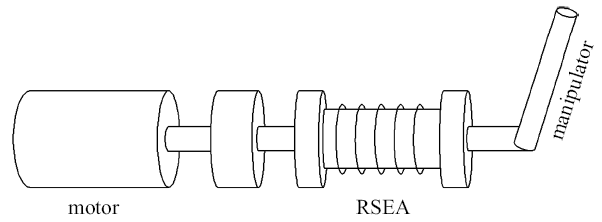Fig. 1   Structure of space manipulator

### 图2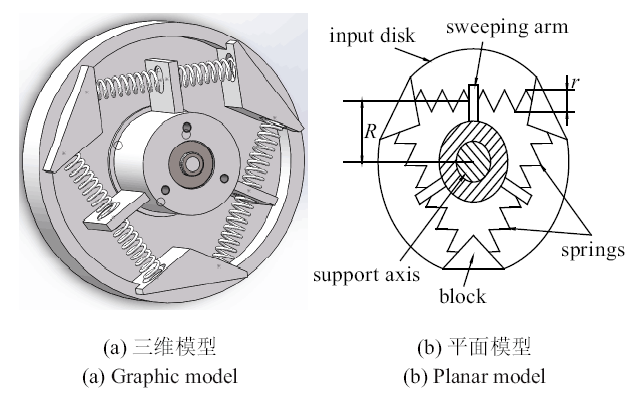Fig. 2   Structure of RSEA

## 2 动力学建模及冲击效应分析

### 图3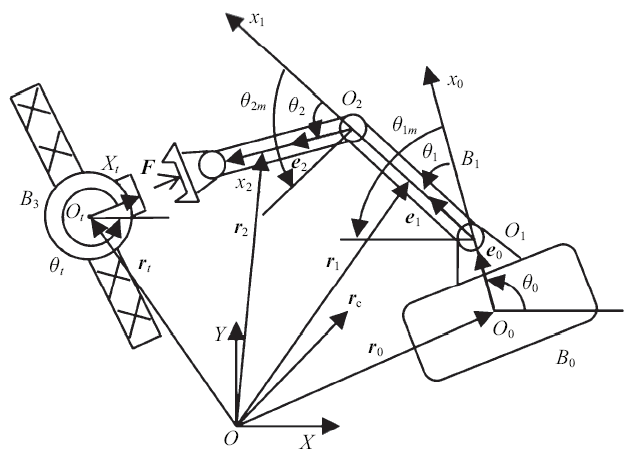Fig. 3   Space robot and target satellite systems

$\begin{eqnarray}{D}_t {\ddot{{q}}}_t ={J}_t^{\rm T} {F}'\end{eqnarray}$

${r}_0 =(x_a \ \ y_a)^{\rm T}$
${r}_i ={r}_0 +\sum\limits_{j=0}^{i-1} {l_i } {e}_i +d_i {e}_i\ \ (i=1,2)$

$\begin{eqnarray} T=\sum\limits_{i=0}^2 (\frac{1}{2} m_i \dot{{r}}_i^2 +\frac{1}{2}I_i {\omega }_i^2 )+\sum\limits_{j=1}^2 {\frac{1}{2}I_{jm} {\omega }_{jm}^2} \end{eqnarray}$

$\begin{eqnarray}U=\sum\limits_{i=1}^2 {\frac{3}{2}} k_{i\rm a} (\Delta x_{i\rm L}^2 +\Delta x_{i\rm R}^2)\end{eqnarray}$

$\begin{eqnarray} \left.\begin{array}{l} D({q}){\ddot{{q}}}+{H}({q},{\dot{{q}}}){\dot{{q}}}={\tau }_{\rm c} +{J}^{\rm T}{F}\\ {J}_m \ddot{\theta}_m +{K}_m ({\theta }_m -{\theta })={\tau}_m\\ {K}_m ({\theta }_m -{\theta })={\tau }_\theta \\ \end{array}\right\} \end{eqnarray}$

$\begin{eqnarray}{D}({q}){\ddot{{q}}}+{H}({q},{\dot{{q}}}){\dot{{q}}}={\tau }_{\rm c} -{J}^{\rm T}({J}_t^{\rm T} )^{-1}{D}_t {\ddot{{q}}}_t\end{eqnarray}$

${D}({q})({\dot{{q}}}(t_0 +\Delta t)-{\dot{{q}}}(t_0 ))+ {J}^{\rm T}({J}_t^{\rm T} )^{-1}{D}_t ({\dot{{q}}}_t (t_0+\Delta t)-{\dot{{q}}}_t (t_0 ))=0$

$\begin{eqnarray}{J\dot{{q}}}={J}_t {\dot{{q}}}_t\end{eqnarray}$

$\begin{eqnarray}{\dot{{q}}}(t_0 +\Delta t)={L}^{-1}[{D}({q}){\dot{{q}}}(t_0 )+{J}^{\rm T}({J}_t^{\rm T} )^{-1}{D}_t {\dot{{q}}}_t(t_0 )]\end{eqnarray}$

$\begin{eqnarray}{D}({q})({\dot{{q}}}(t_0 +\Delta t)-{\dot{{q}}}(t_0 ))={J}^{\rm T}{P}\end{eqnarray}$

${P}=({J}^{\rm T}\mbox{)}^{+1}{D}({q})[{L}^{-1}({D}({q}){\dot{{q}}}(t_0 )+ {J}^{\rm T}({J}_t^{\rm T} )^{-1}{D}_t {\dot{{q}}}_t(t_0 ))-{\dot{{q}}}(t_0 )]$

$\begin{eqnarray}{F}=\frac{{P}}{\Delta t}\end{eqnarray}$

$\begin{eqnarray}{\ddot{{q}}}_t ={J}_t^{-1} [{J\ddot{{q}}}+({\dot{{J}}}-{\dot{{J}}}_t {J}_t^{-1} {J}){\dot{{q}}}]\end{eqnarray}$

$\begin{eqnarray} \left. \begin{array}{l} {D}_{\rm C} ({q}){\ddot{{q}}}+{H}_{\rm C} ({q},{\dot{{q}}}){\dot{{q}}}={\tau}_{\rm c}\\ {J}_m {\ddot{{\theta }}}_m +{K}_m ({\theta }_m -{\theta })={\tau }_m\\ {K}_m ({\theta }_m -{\theta })={\tau }_\theta \\ \end{array}\right\} \end{eqnarray}$

$\begin{eqnarray} \left[ {{\begin{array}{ll} {{D}_{\rm C11} } & {{D}_{\rm C12} } \\ {{D}_{\rm C21} } & {{D}_{\rm C22} } \\ \end{array} }} \right]\left[ {{\begin{array}{l} {{\ddot{{q}}}_a } \\ {{\ddot{{q}}}_\theta } \\ \end{array} }} \right]+\left[ {{\begin{array}{ll} {{H}_{\rm C11} } & {{H}_{\rm C12} } \\ {{H}_{\rm C21} } & {{H}_{\rm C22} } \\ \end{array} }} \right]\left[ {{\begin{array}{l} {{\dot{{q}}}_a } \\ {{\dot{{q}}}_\theta } \\ \end{array} }} \right]=\left[ {{\begin{array}{l} {{\tau }_a } \\ {{\tau }_b } \\ \end{array} }} \right] \end{eqnarray}$

$\begin{eqnarray} \left.\begin{array}{l} {{D}_X {\ddot{{q}}}_\theta +{H}_X {\dot{{q}}}_\theta ={\tau }_b}\\ {{J}_m {\ddot{{\theta }}}_m +{K}_m ({\theta }_m -{\theta })={\tau }_m }\\ {{K}_m ({\theta }_m -{\theta })={\tau }_\theta }\\ \end{array}\right\} \end{eqnarray}$

## 3 控制器设计

### 3.1 快变子系统控制器设计

$\begin{eqnarray}{\tau }_m ={\tau }_{\rm s} +{\tau }_{\rm f}\end{eqnarray}$

$\begin{eqnarray}{K}_m =\frac{{K}_1 }{\varepsilon ^2}\end{eqnarray}$

$\begin{eqnarray}\varepsilon ^2{\ddot{{\tau }}}_\theta ={J}_m^{-1} {K}_1 ({\tau }_m -{J}_m {\ddot{{\theta }}}-{\dot{{\tau }}}_\theta )\end{eqnarray}$

$\begin{eqnarray} {\tau }_{\rm f} =-{K}_{\rm f} ({\dot{{\theta }}}_m -{\dot{{\theta }}}) \end{eqnarray}$

$\begin{eqnarray} \varepsilon ^2{J}_m {\ddot{{\tau }}}_\theta ={K}_1 ({\tau }_{\rm s} -{J}_m {\ddot{{\theta }}}-{\tau }_\theta )-\varepsilon {K}_{\rm 2} {\dot{{\tau }}}_\theta \end{eqnarray}$

$\begin{eqnarray}{D}_{S\theta } {\ddot{{q}}}_\theta +{H}_{S\theta } {\dot{{q}}}_\theta ={\tau }_{S\theta }\end{eqnarray}$

### 3.2 慢变子系统控制器设计

$\begin{eqnarray}{D}_{S\theta } {\ddot{{q}}}_\theta +{H}_{S\theta } {\dot{{q}}}_\theta ={\tau }_{S\theta } +{\tau }_{\rm d}\end{eqnarray}$

$\begin{eqnarray} \left.\begin{array}{l} {{\dot{{x}}}_1 ={x}_2}\\ {{\dot{{x}}}_2 =-{Ax}_2 +{B}+{x}_3 }\\ \end{array} \right\} \end{eqnarray}$

(1) 定义轨迹跟踪误差, 即第一个误差面

$\begin{eqnarray}{s}_1 ={e}={x}_1 -{q}_{\theta\rm d}\end{eqnarray}$

$\begin{eqnarray}{\dot{{s}}}_1 ={x}_2 -{\dot{{q}}}_{\theta\rm d}\end{eqnarray}$

$\begin{eqnarray}{\bar{{x}}}_2 =-\eta _1 {s}_1 +{\dot{{q}}}_{\theta\rm d}\end{eqnarray}$

$\begin{eqnarray} \left.\begin{array}{l} {\eta _2 {\dot{{x}}}_{\rm 2d} +{x}_{\rm 2d} ={\bar{{x}}}_2 }\\ {{x}_{\rm 2d} (0)={\bar{{x}}}_2 (0)} \\ \end{array}\right\} \end{eqnarray}$

(2) 为设计慢变子系统控制律, 定义第二个误差面

$\begin{eqnarray}{s}_2 ={x}_2 -{x}_{\rm 2d}\end{eqnarray}$

$\begin{eqnarray}{\dot{{s}}}_2 =-{D}_{S\theta }^{-1} {H}_{S\theta } {x}_2 +{D}_{S\theta }^{-1} {\tau }_{S\theta } +{x}_3 -{\dot{{x}}}_{\rm 2d}\end{eqnarray}$

$\begin{eqnarray} \left. \begin{array}{l} {{\dot{{\hat{{x}}}}}_1 ={\hat{{x}}}_2 +{\alpha }_1 {\tilde{{x}}}_1} \\ {{\dot{{\hat{{x}}}}}_2 ={\hat{{x}}}_3 +{D}_{S\theta }^{-1} ({\tau }_{S\theta } -{H}_{S\theta } {\dot{{q}}}_\theta )+{\alpha }_2 {\tilde{{x}}}_1}\\ {{\dot{{\hat{{x}}}}}_3 ={\alpha }_3 {\tilde{{x}}}_1}\\ \end{array}\right\} \end{eqnarray}$

$\begin{eqnarray}{\alpha }_1 =3\lambda {I}_3 , \ \ {\alpha }_2 =3\lambda ^2{I}_3 , \ \ {\alpha }_3 =\lambda ^3{I}_3\end{eqnarray}$

$\begin{eqnarray}{\tau }_{S\theta } ={D}_{S\theta } ({\dot{{x}}}_{\rm 2d} -{c}_2 {s}_2 )+{H}_{S\theta } {x}_2 -{D}_{S\theta } {\hat{{x}}}_3\end{eqnarray}$

$\begin{eqnarray}{\dot{{\omega }}}=\lambda {K}_v {\omega }+\frac{{K}_p {\dot{{x}}}_3 (t)}{\lambda ^2}\end{eqnarray}$

${\omega }(t)=\int_0^t {{\rm e}^{\lambda {K}_v (t-\tau )}} \frac{{K}_p {\dot{{x}}}_3 (\tau )}{\lambda ^2}{\rm d}\tau = \\ \qquad\frac{1}{\lambda ^2}[\int_0^t {\lambda {K}_v {\rm e}^{\lambda {K}_v (t-\tau )}} {K}_p {x}_3 (\tau ){\rm d}\tau +{K}_p {x}_3 (t)]$

${\omega }(t)\leqslant \frac{1}{\lambda ^2}[\int_0^t {\lambda {K}_v {\rm e}^{\lambda {K}_v (t-\tau )}} {K}_p {x}_{\rm M} {\rm d}\tau +{K}_p {x}_{\rm M}]=\\ \qquad \frac{1}{\lambda ^2}{\rm e}^{\lambda {K}_v t}{K}_p {x}_{\rm M}$

$\begin{eqnarray}{\vert}[{\rm e}^{\lambda {K}_v t}]_{ij} {\vert}\leqslant \frac{1}{\lambda ^9}\ \ \ (i,j=1,2,\cdots ,9)\end{eqnarray}$

$\begin{eqnarray}{\vert }[{\omega }_{ij} (t)]{\vert }\leqslant \frac{[{x}_{\rm M} ]_i }{\lambda ^{11}}\ \ \ (i,j=1,2,3)\end{eqnarray}$

$\begin{eqnarray}\vert {\tilde{{x}}}_{ij} \vert \leqslant \frac{[{x}_{\rm M} ]_i }{\lambda ^{12-i}}\leqslant \gamma _i\ \ \ (i,j=1,2,3)\end{eqnarray}$

$\begin{eqnarray}{z}_2 ={x}_{\rm 2d} -{\bar{{x}}}_2\end{eqnarray}$

$\begin{eqnarray}{\dot{{x}}}_{\rm 2d} =-{z}_2 /\eta _2\end{eqnarray}$

${\dot{{z}}}_2 =-{z}_2 /\eta _2 -\eta _1 {\dot{{s}}}_1 +{\ddot{{q}}}_{\theta\rm d} = -{z}_2 /\eta _2 +{ \varPhi }({s}_1 ,{s}_2 ,{q}_{\theta\rm d},{\dot{{q}}}_{\theta\rm d} ,{\ddot{{q}}}_{\theta\rm d} ,{z}_2 )$

$\begin{eqnarray}{\dot{{s}}}_1 ={s}_2 +{z}_2 -\eta _1 {s}_1\end{eqnarray}$

$\begin{eqnarray} V=\frac{1}{2}({s}_1^{\rm T} {s}_1 +{s}_2^{\rm T} {s}_2 +{z}_2^{\rm T} {z}_2 ) \end{eqnarray}$

$\begin{eqnarray}\dot{{V}}={s}_1^{\rm T} {\dot{{s}}}_1 +{s}_2^{\rm T} {\dot{{s}}}_2 +{z}_2^{\rm T} {\dot{{z}}}_2\end{eqnarray}$

$\begin{eqnarray}{\dot{{s}}}_2 =-{c}_2 {s}_2 +{\tilde{{x}}}_3\end{eqnarray}$

$\dot{{V}}={s}_1^{\rm T} ({s}_2 +{z}_2 -\eta _1 {s}_1 )+{s}_2^{\rm T} (-{c}_2 {s}_2 +{\tilde{{x}}}_3 )+ \\ \qquad{z}_2^{\rm T} (-{z}_2 /\eta _2 +{ \varPhi }({s}_1,{s}_2,{q}_{\theta\rm d} ,{\dot{{q}}}_{\theta\rm d} ,{\ddot{{q}}}_{\theta\rm d},{z}_2 ))$

$\left\{\begin{array}{l} {s}_1^{\rm T} {s}_2 \leqslant \dfrac{1}{2}{s}_1^{\rm T} {s}_1 +\dfrac{1}{2}{s}_2^{\rm T} {s}_2 \\ {s}_1^{\rm T} {z}_2 \leqslant \dfrac{1}{2}{s}_1^{\rm T} {s}_1 +\dfrac{1}{2}{z}_2^{\rm T} {z}_2 \\ {s}_2^{\rm T} {\tilde{{x}}}_3 \leqslant \dfrac{1}{2}{s}_2^{\rm T} {s}_2 +\dfrac{1}{2}{\tilde{{x}}}_3^{\rm T} {\tilde{{x}}}_3 \\ {z}_2^{\rm T} { \varPhi }({s}_1 ,{s}_2 ,{q}_{\theta\rm d},{\dot{{q}}}_{\theta\rm d} ,{\ddot{{q}}}_{\theta\rm d} ,{z}_2 )\leqslant \dfrac{{z}_2^{\rm T} {z}_2 { \varPhi }^{\rm T}{ \varPhi}}{2k}+\dfrac{k}{2} \end{array}\right.$

$\dot{{V}}\leqslant -{s}_1^{\rm T} \eta _1 {s}_1 -{c}_2 {s}_2^{\rm T} {s}_2 -{z}_2^{\rm T} {z}_2 /\eta _2 +{s}_2^{\rm T} {s}_2 + \\ \quad \frac{1}{2}{z}_2^{\rm T} {z}_2 +\frac{1}{2}{\tilde{{x}}}_3^{\rm T} {\tilde{{x}}}_3 +\frac{{z}_2^{\rm T} {z}_2 { \varPhi }^{\rm T}{ \varPhi }}{2k}+\frac{k}{2}\\ \quad \leqslant (1-\eta _1 ){s}_1^{\rm T} {s}_1 +(1-{c}_2 ){s}_2^{\rm T} {s}_2 +\\ \quad {z}_2^{\rm T} (\frac{1}{2}-\frac{1}{\eta _2 }+\frac{{ \varPhi }^{\rm T}{ \varPhi }}{2k}){z}_2 +\frac{1}{2}{\tilde{{x}}}_3^{\rm T} {\tilde{{x}}}_3 +\frac{k}{2}$

$\begin{eqnarray}\dot{{V}}\leqslant -\varsigma V+\rho\end{eqnarray}$

$\begin{eqnarray}0\leqslant V\leqslant \rho /\varsigma +[V(0)-\rho /\varsigma ]{\rm e}^{-\varsigma t}\end{eqnarray}$

## 4 仿真算例分析

### 4.1 捕获碰撞过程RSEA抗冲击性能模拟

$\begin{eqnarray}{K}_m =2{K}_a (3R^2+r^2)(2\cos ^2{\varphi }-1)\end{eqnarray}$

Table 1  RSEA impact resistance at different satellite initial velocities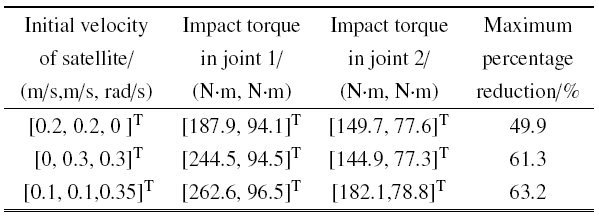### 图4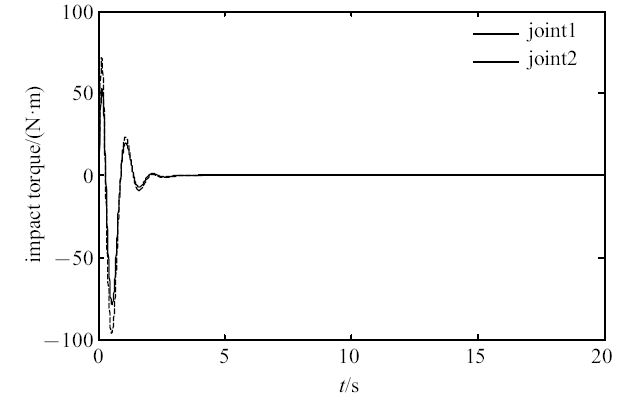Fig. 4   Joint impact torque without switching strategy

### 图5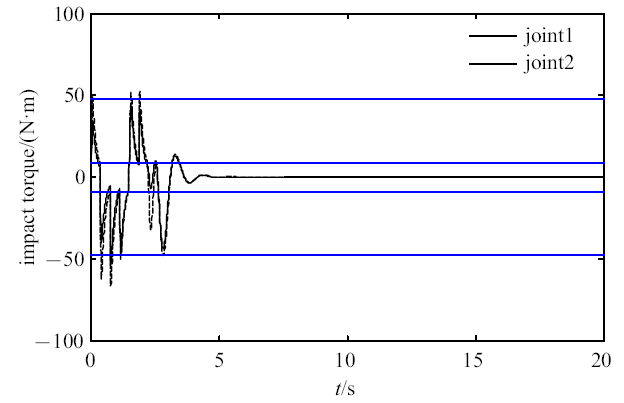Fig. 5   Joint impact torque with switching strategy

### 图6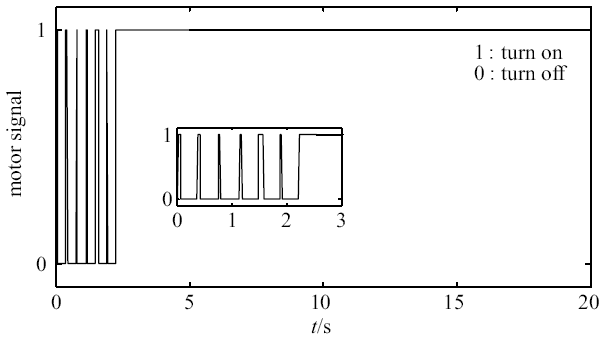Fig. 6   Switch signal of joint motor

### 图7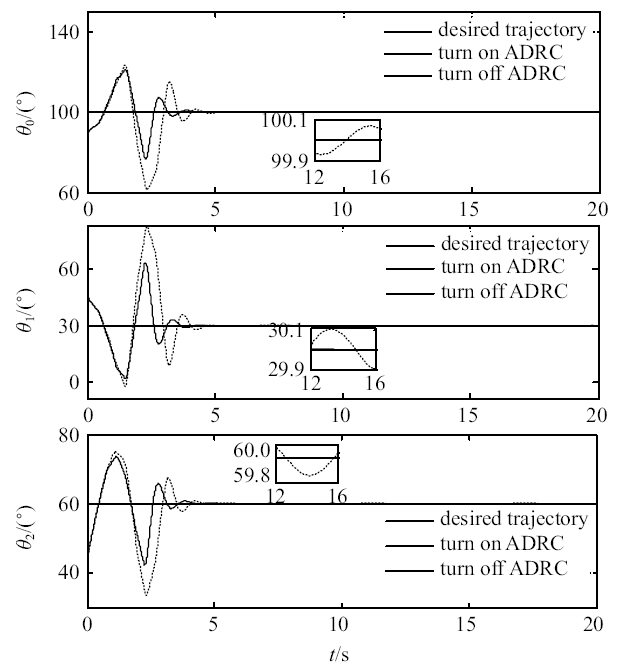Fig. 7   Trajectory tracking of stabilization under buffer and compliant control

### 图8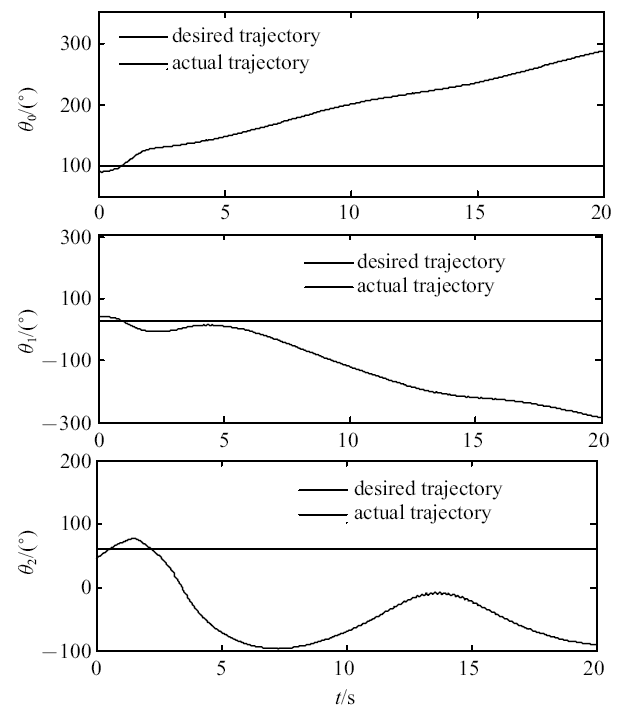Fig. 8   Trajectory without fast controller

## 参考文献 原文顺序 文献年度倒序 文中引用次数倒序 被引期刊影响因子

Meng QL, Liang JX, Ma O.

Identification of all the inertial parameters of a non-cooperative object in orbit

Aerospace Science and Technology, 2019,91:571-582

URL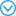Based on the Legendre pseudospectral method, the optimal control of free floating space robots path planning problems are studied. Free floating is the working status for the space robots in task and path planning is the foundation for them to fulfil a complex space task. Because the space robots have no fixed pedestal and there are nonholonomic constraints between the manipulator and the carrier, and it makes the path planning for free floating space robots different from those on the ground. In this paper, the Legendre pseudospectral method which can realize the optimal control of free floating space robots path planning problem is presented. Firstly, a dynamic model of free floating space robots is established by using multi-body dynamics theory. The path planning problem of Bolza with certain initial and terminal stance is then obtained. Here, we select the minimum joint dissipation as performance index and consider the actual control input saturation. Then, by applying the Legendre pseudospectral method, the state and control variables are discretized at Legendre-Gauss-Lobatto (LGL) point and Lagrange interpolation polynomials are constructed to approximate the state and control variables. The problem of continuous path optimization is discretized to solve a nonlinear programming problem. Finally, results of the numerical simulation show that using the application of Legendre pseudospectral method to solve the problem of free floating space robot path planning can get the optimal trajectory of manipulator and carrier. It can fit various constraint conditions well. And this method is with fast simulation calculation, high accuracy and good real-time performance.

( Ge Xinsheng, Chen Kaijie.

Path planning of free floating space robot using Legendre pseudospectral method

Chinese Journal of Theoretical and Applied Mechanics, 2016,48(4):823-831 (in Chinese))

URLBased on the Legendre pseudospectral method, the optimal control of free floating space robots path planning problems are studied. Free floating is the working status for the space robots in task and path planning is the foundation for them to fulfil a complex space task. Because the space robots have no fixed pedestal and there are nonholonomic constraints between the manipulator and the carrier, and it makes the path planning for free floating space robots different from those on the ground. In this paper, the Legendre pseudospectral method which can realize the optimal control of free floating space robots path planning problem is presented. Firstly, a dynamic model of free floating space robots is established by using multi-body dynamics theory. The path planning problem of Bolza with certain initial and terminal stance is then obtained. Here, we select the minimum joint dissipation as performance index and consider the actual control input saturation. Then, by applying the Legendre pseudospectral method, the state and control variables are discretized at Legendre-Gauss-Lobatto (LGL) point and Lagrange interpolation polynomials are constructed to approximate the state and control variables. The problem of continuous path optimization is discretized to solve a nonlinear programming problem. Finally, results of the numerical simulation show that using the application of Legendre pseudospectral method to solve the problem of free floating space robot path planning can get the optimal trajectory of manipulator and carrier. It can fit various constraint conditions well. And this method is with fast simulation calculation, high accuracy and good real-time performance.

URL( Zhang Dingguo.

Recursive Lagrangian dynamic modeling and simulation of multi-link spatial flexible manipulator arms

Applied Mathematics and Mechanics, 2009,30(10):1202-1212 (in Chinese))

URLYu XY, Chen L.

Observer-based two-time scale robust control of free-flying flexible-joint space manipulators with external disturbances

Robotica, 2017,35(11):1-17

Ai HP, Chen L.

Variable structure sliding mode control based on passivity theory for free floating space robot

// The 69th International Astronautical Congress, Bremen, Germany, 2018

Virgili-Llop J, Zagaris C, Zappulla R, et al.

A convex-programming-based guidance algorithm to capture a tumbling object on orbit using a spacecraft equipped with a robotic manipulator

The International Journal of Robotics Research, 2019,38(1):40-72

Liu XF, Li HQ, Cai GP, et al.

Dynamics analysis of flexible space robot with joint friction

Aerospace Science and Technology, 2015,47:164-176

( Yuan Changqing, Li Junfeng, Wang Tianshu, et al.

An optimal and robust attitude-tracking control of spacecraft based on inverse system method

Engineering Mechanics, 2008,25(2):214-218 (in Chinese))

Nonlinear attitude control of spacecraft with a large captured object

Journal of Guidance, Control, and Dynamic, 2016,39(4):754-769

( Zhao Hang, Zhao Yang, Tian Hao, et al.

Key techniques and applications of space cellular robotic system

Journal of Astronautics, 2018,39(10):1071-1080 (in Chinese))

Lim J, Chung J.

Dynamic analysis of a tethered satellite system for space debris capture

Nonlinear Dynamics, 2018,94:1-18

( Sun Jialiang, Tian Qiang, Hu Haiyan.

Advances in dynamic modeling and optimization of flexible multibody systems

Chinese Journal of Theoretical and Applied Mechanics, 2019,51(6):1565-1586 (in Chinese))

( Fu Xiaodong, Chen Li.

An input limited repetitive learning control of flexible-base two-flexible-link and two-flexible-joint space robot with integration of motion and vibration

Chinese Journal of Theoretical and Applied Mechanics, 2020,52(1):171-183 (in Chinese))

( Zhu An, Chen Li.

Mechanical simulation and full order sliding mode collision avoidance compliant control based on neural network of dual-arm space robot with compliant mechanism capturing satellite

Chinese Journal of Theoretical and Applied Mechanics, 2019,51(4):1156-1169 (in Chinese))

Shah S V, Sharf I, Misra A.

Reactionless path planning strategies for capture of tumbling objects in space using a dual-arm robotic system

// AIAA Guidance, Navigation, and Control Conference, Boston, USA, 2013: 4521

( Liu Shuai, Wu Shunan, Liu Yufei, et al.

Autonomous reinforcement learning control for space robot to capture non-cooperative targets

Scientia Sinica Physica,Mechanica & Astronomica, 2019,49(2):113-122 (in Chinese))

Wang MM, Luo JJ, Yuan JP, et al.

Detumbling strategy and coordination control of kinematically redundant space robot after capturing a tumbling target

Nonlinear Dynamics, 2018,92(3):1023-1043

Boning P, Dubosky S.

A kinematic approach to determining the optimal actuator sensor architecture for space robots

The International Journal of Robotics Research, 2011,30(9):1194-1204

URLAs the technology of space science develops rapidly, space robot system is expected to capture the noncooperative satellite on-orbit. Space robot with dual-arm obviously has more comparative advantage in this respect compared with the one with single arm. Because of the complicated condition in outer space it makes the dynamics and control problems related to satellite-capturing operation by space robot system with dual-arm to be extremely complicated, and there are some unique characteristics, such as, nonholonomic dynamics restriction, change of system configuration, transfer of linear momentum, angular momentum and energy, topology transfer from open to closed loop system, and the constraints of closed-loop geometry and kinematics during satellite-capturing operation. In this paper, the dynamic evolution for space robot with dual-arm capturing a spin satellite and calm control for unstable closed chain composite system are discussed. At first, with the Lagrangian approach, the dynamic model of open chain space robot with dual-arm before capture operation is established, and dynamic model of satellite is derived by Newton-Euler method. On that basis, based on the law of conservation of momentum and the law of force transfer, the impact effect after collision of space robot with dual-arm to capture the target is analyzed and solved by the process of integration and simplification, and the suitable capture operation strategy is given. Closed chain constraint equations are obtained by the constraints of closed-loop geometry and kinematics of closed chain system. With the closed chain constraint equations, the composite system dynamic model is derived. For the unstable closed chain composite system after the capture, the fuzzy H control scheme for calm motion is designed. The fuzzy logic system is applied to overcome the influence of uncertainty part and the robust H control item is used to eliminate the approximate error, to guarantee the tracking precision. The global stability of the system is proved by the Lyapunov theory. The weighted minimum-norm theory is introduced to distribute torques guaranteeing that the cooperative operation between manipulators. At last, numerical examples simulated the response of collision are used to verify the efficiency of the control scheme.

( Cheng Jing, Chen Li.

Mechanical analysis and calm control of dual-arm space robot for capturing a satellite

Chinese Journal of Theoretical and Applied Mechanics, 2016,48(4):832-842 (in Chinese))

URLAs the technology of space science develops rapidly, space robot system is expected to capture the noncooperative satellite on-orbit. Space robot with dual-arm obviously has more comparative advantage in this respect compared with the one with single arm. Because of the complicated condition in outer space it makes the dynamics and control problems related to satellite-capturing operation by space robot system with dual-arm to be extremely complicated, and there are some unique characteristics, such as, nonholonomic dynamics restriction, change of system configuration, transfer of linear momentum, angular momentum and energy, topology transfer from open to closed loop system, and the constraints of closed-loop geometry and kinematics during satellite-capturing operation. In this paper, the dynamic evolution for space robot with dual-arm capturing a spin satellite and calm control for unstable closed chain composite system are discussed. At first, with the Lagrangian approach, the dynamic model of open chain space robot with dual-arm before capture operation is established, and dynamic model of satellite is derived by Newton-Euler method. On that basis, based on the law of conservation of momentum and the law of force transfer, the impact effect after collision of space robot with dual-arm to capture the target is analyzed and solved by the process of integration and simplification, and the suitable capture operation strategy is given. Closed chain constraint equations are obtained by the constraints of closed-loop geometry and kinematics of closed chain system. With the closed chain constraint equations, the composite system dynamic model is derived. For the unstable closed chain composite system after the capture, the fuzzy H control scheme for calm motion is designed. The fuzzy logic system is applied to overcome the influence of uncertainty part and the robust H control item is used to eliminate the approximate error, to guarantee the tracking precision. The global stability of the system is proved by the Lyapunov theory. The weighted minimum-norm theory is introduced to distribute torques guaranteeing that the cooperative operation between manipulators. At last, numerical examples simulated the response of collision are used to verify the efficiency of the control scheme.

URLThis paper discusses the collision dynamics modeling of a free-floating space-based robot in the process of on-orbit satellite capturing, and it also analyzes the stability control for the compounded body of the space-based robot and target after the capturing operation is completed. First the method of multibody system dynamics modeling is employed in combination with the characteristics of collision dynamics of the space-based robot while it captures the target satellite to set up a dynamics model for the free-floating space-based robot during its on-orbit capture of the floating satellite. Based on it, the movement speed for the compounded body of the space-based robot and target is calculated. In view of the fact that for the inertia parameters of both the satellite and the space-based robot system are unknown, the model and the theory of neural network control and Lyapunov stability theory are used to design a Gaussian radial basis function neural network control scheme for the compounded body to move stably under the influence of collision and impact during the capturing process. Thus, the effective control is realized for capturing a target satellite. The Gaussian radial basis function neural network control scheme has the obvious advantages of requiring no feedback and measurement of the position,velocity,acceleration,attitude angle velocity and attitude angle acceleration of the floating base. Finally, the validity and applicability of the control scheme are manifested by system numerical simulation.

( Liang Jie, Chen Li.

Dynamics modeling for free-floating space-based robot during satellite capture and RBF neural network control for compound body sable movement

Acta Aeronautica et Astronautica Sinica, 2013,34(4):970-978 (in Chinese))

URLThis paper discusses the collision dynamics modeling of a free-floating space-based robot in the process of on-orbit satellite capturing, and it also analyzes the stability control for the compounded body of the space-based robot and target after the capturing operation is completed. First the method of multibody system dynamics modeling is employed in combination with the characteristics of collision dynamics of the space-based robot while it captures the target satellite to set up a dynamics model for the free-floating space-based robot during its on-orbit capture of the floating satellite. Based on it, the movement speed for the compounded body of the space-based robot and target is calculated. In view of the fact that for the inertia parameters of both the satellite and the space-based robot system are unknown, the model and the theory of neural network control and Lyapunov stability theory are used to design a Gaussian radial basis function neural network control scheme for the compounded body to move stably under the influence of collision and impact during the capturing process. Thus, the effective control is realized for capturing a target satellite. The Gaussian radial basis function neural network control scheme has the obvious advantages of requiring no feedback and measurement of the position,velocity,acceleration,attitude angle velocity and attitude angle acceleration of the floating base. Finally, the validity and applicability of the control scheme are manifested by system numerical simulation.

Uyama N, Hirano D, Nakanishi H, et al.

Impedance-based contact control of a free-flying space robot with respect to coefficient of restitution

// IEEE/SICE International Symposium on System Integration, Kyoto, Japan, IEEE, 2012: 1196-1201

On-orbit cooperating space robotic servicers handling a passive object

IEEE Transactions on Aerospace and Electronic Systems, 2015,51(2):802-814

Meng DS, Lu WN, Xu WF, et al.

Vibration suppression control of free-floating space robots with flexible appendages for autonomous

Acta Astronautica, 2018,151:904-918

Aghili F.

Optimal control of a space manipulator for detumbling of a target satellite

// IEEE International Conference on Robotics and Automation, Kobe, Japan: IEEE, 2009: 3019-3024

Dong H, Hu Q, Akella MR.

Dual-Quaternion-based spacecraft autonomous rendezvous and docking under six-degree-of-freedom motion constraints

Journal of Guidance, Control, and Dynamics, 2018,41(5):1150-1162

Lampariello R, Hirzinger G.

Generating feasible trajectories for autonomous on-orbit grasping of spinning debris in a useful time

// IEEE/RSJ International Conference on Intelligent Robots and Systems, Tokyo, Japan, IEEE, 2013: 5252-5259

Stolfi A, Gasbarri P, Sabatini M.

A combined impedance-PD approach for controlling a dual-arm space manipulator in the capture of a non-cooperative target

Acta Astronautica, 2017,139:243-253

Gu X, Wang K, Cheng T, et al.

Mechanical design of a 3-DOF humanoid soft arm based on modularized series elastic actuator

// IEEE International Conference on Mechatronics and Automation, Beijing, China. IEEE, 2015: 1127-1131

Calanca A, Fiorini P.

Understanding environment- adaptive force control of series elastic actuators

IEEE/ASME Transactions on Mechatronics, 2018,23(1):413-423

( Han Jinqing.

Auto-disturbances-rejection controller and its applications

Control and Decision, 1998,13(1):19-23 (in Chinese))

Arcos-Legarda J, Cortes-Romero J, Beltran-Pulido A, et al.

Hybrid disturbance rejection control of dynamic bipedal robots

Multibody System Dynamics, 2019,46(3):281-306

Swaroop D, Hedrick JK, Yip PP, et al.

Dynamic surface control for a class of nonlinear systems

IEEE Transactions on Automatic Control, 2000,45(10):1893-1899

Von E Karl D.

Dynamic surface control of trajectory tracking marine vehicles with actuator magnitude and rate limits

Automatica, 2019,105:433-442

Gao ZQ.

Scaling and bandwidth-parameterization based controller tuning

// Proceedings of the American Control Conference, Denver, USA: IEEE, 2003: 4989-4996

Qing Z, Linda Q.

On Stability analysis of active disturbance rejection control for nonlinear time-varying plants with unknown dynamics

// IEEE Conference on Decision and Control, New Orleans, USA. IEEE, 2007: 12-14

Chen WS.

Adaptive backstepping dynamic surface control for systems with periodic disturbances using neural networks

IET Control Theory and Applications, 2009,3(10):1383-1394

/

 〈〉